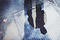# The Union-Find Data Structure in Swift

## That is, disjoint setsImage by Giorgio Trovato

# Before we start

Difficulty: Beginner | Easy | Normal | Challenging
This article has been developed using Xcode 12.1, and Swift 5.3

* You will be expected to be aware how to either make a Single View Application in Swift or use a Playground
* It would be useful to have some knowledge of Sets in Swift
* A dictionary is used to store the nodes

Data Structure: A way of formatting data Disjoint: (of two or more sets) having no elements in common

Union-find data structure: A data structure that sorts a collection of disjoint sets

# The Union-Find Data

Disjoint-set data structures play a key role in an efficient version of Kruskal’s algorithm for finding the minimal spanning tree of a graph, and the data structure form the basis of a wide variety of algorithms including cycle detection.

More than that, they are have applications in Data Science and are even commonly used for LeetCode questions.

Depending on your needs, they can be a big deal.

If we have two sets that do not share elements we have a situation like that shown in the diagram above.

In Swift this might be represented as the following sets, p and q.

That is, the sets p and q are disjoint because they have no members in common. The example works with any type of set you would like to implement in Swift.

Equality is transitive. This means:

`if a = b and b = c, then a = c`

We can perform a BFS from any two nodes — if one node can be reached from the other they are by definition in the same set.

By adopting this approach, a BFS would have to be performed for each query (that is, each pair you wish to test whether they are in the same set) leaving the time complexity as O(N * M) where N is the set size and M is total number of queries to be tested.

An alternative is to use the `Union-Find Data Structure` where elements in the same set are kept in a single group. It is this approach that this article will focus on.

This node must be a `class` type as the node references itself. The class also confoms to the Hashable protocol

Main operations There are three basic operations that are used in such a data structure:

• find: Determine which subset an element is within
• union: Join two subsets into a single set
• addSet: Create a new set with an element

Therefore to create a tree (which effectively the set is) we will need to combine `addSet` and `union` since every time we wish to add a new element we must first call `addSet` to add it, before using `union` to join it into an existing set.

This means we might have the set {“a”, “b”} and then add a set {“c”}.<br<

We then can call union on two elements for example

So each subset is represented by a tree.

The UnionFind class Here we conform to the Hashable protocol and the Comparable protocol, and store an index of nodes and a set number for each one.

As a result the class is defined as follows:

with, of course, the following functions are declared below:

addSet

Here we create a new tree for each element, and the index is taken from the number of nodes:

find

We can check the index dictionary

union

We take the root for the tree from the firstElement, and the root from the secondElement. Then we put the secondNode onto the firstNode, for example:

sameSet (additional)

If the index for the firstElement is the same as the index for the secondElement, they are in the same set.

Improvements

These improvements come under the banner of heuristics, and enable performance improvesment

Union by Rank

When performing a `union`, if one tree has a shorter depth then ithe algorithm should favour keeping the tree as short as possible. This can be accomplished by moving the longer tree to the shorter one. This can reduce the time to find the root of any particular tree.

Path Compression

All nodes on a tree should have a single parent, which is the root.

Something approaching this has been implemented in my version of the Union-find structure described above, and is shown in the Repo.

# Conclusion

The Repo has all of the code so you can see how this all works in a working Swift Playground.

If you’ve any questions, comments or suggestions please hit me up on Twitter

stevecurtis.me